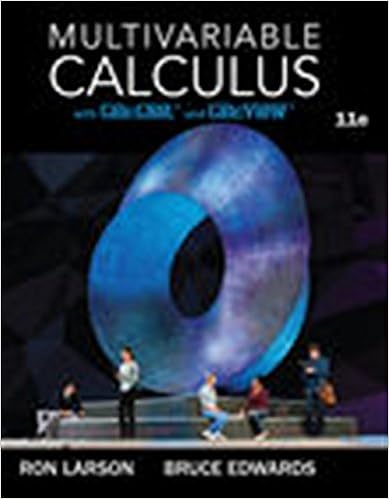# State all simplification rules for cond, and, or 2 Why and,...

• Homework Help
• 2

This preview shows page 1 - 2 out of 2 pages.

##### We have textbook solutions for you!
The document you are viewing contains questions related to this textbook.The document you are viewing contains questions related to this textbook.
Chapter 15 / Exercise 10
Multivariable Calculus
LarsonExpert Verified
CS 135 — Problem Set 2 Winter 2017 [Theory] 1. State all simplification rules for cond, and, or. 2. Why (and), (or) rules are necessary? 3. What is dynamic and static typing? What are pros and cons of each? 4. What is data definition? What is structure definition? 5. What functions are created after structure definition? [Practice] For stepping questions, you may assume that code has been processed in Racket. 1. Produce a step-by-step evaluation of the following program. (make-posn (+ 2 3) (or false false)) 2. Produce a step-by-step evaluation of the following program. (define a 5) (* (/ (- 100 (quotient 30 11) ) 49) (+ a a)) 3. Produce a step-by-step evaluation of the following program. (define x (/ 10 2)) (define y (* x 2)) (define (f x)(sqrt (+ (sqr x) (sqr y)))) (f (+ x y)) 4. Produce a step-by-step evaluation of the following program. (define m (/ 10 2)) (define k (* m 2)) (define (f m k)(and true (cond [(or (positive? m) k) true] [(or (negative? m) k) false]))) (f 0 (and (= m 5) ( > k 11)))
##### We have textbook solutions for you!
The document you are viewing contains questions related to this textbook.The document you are viewing contains questions related to this textbook.
Chapter 15 / Exercise 10
Multivariable Calculus
LarsonExpert Verified
•••